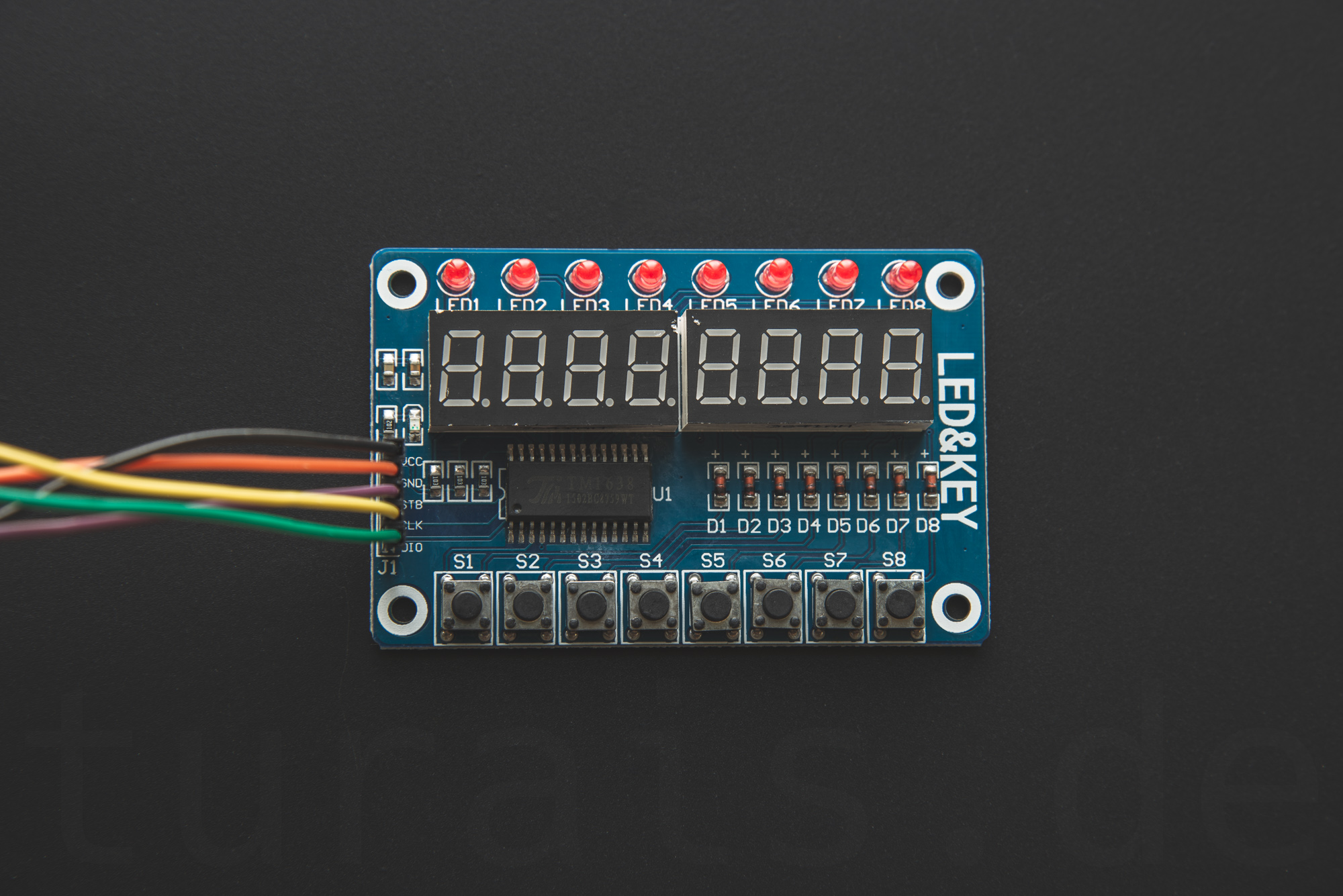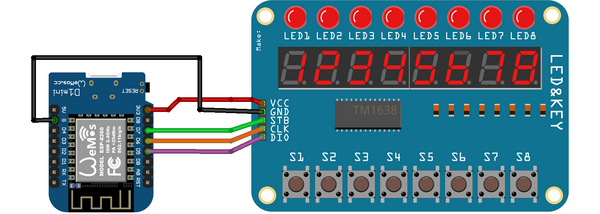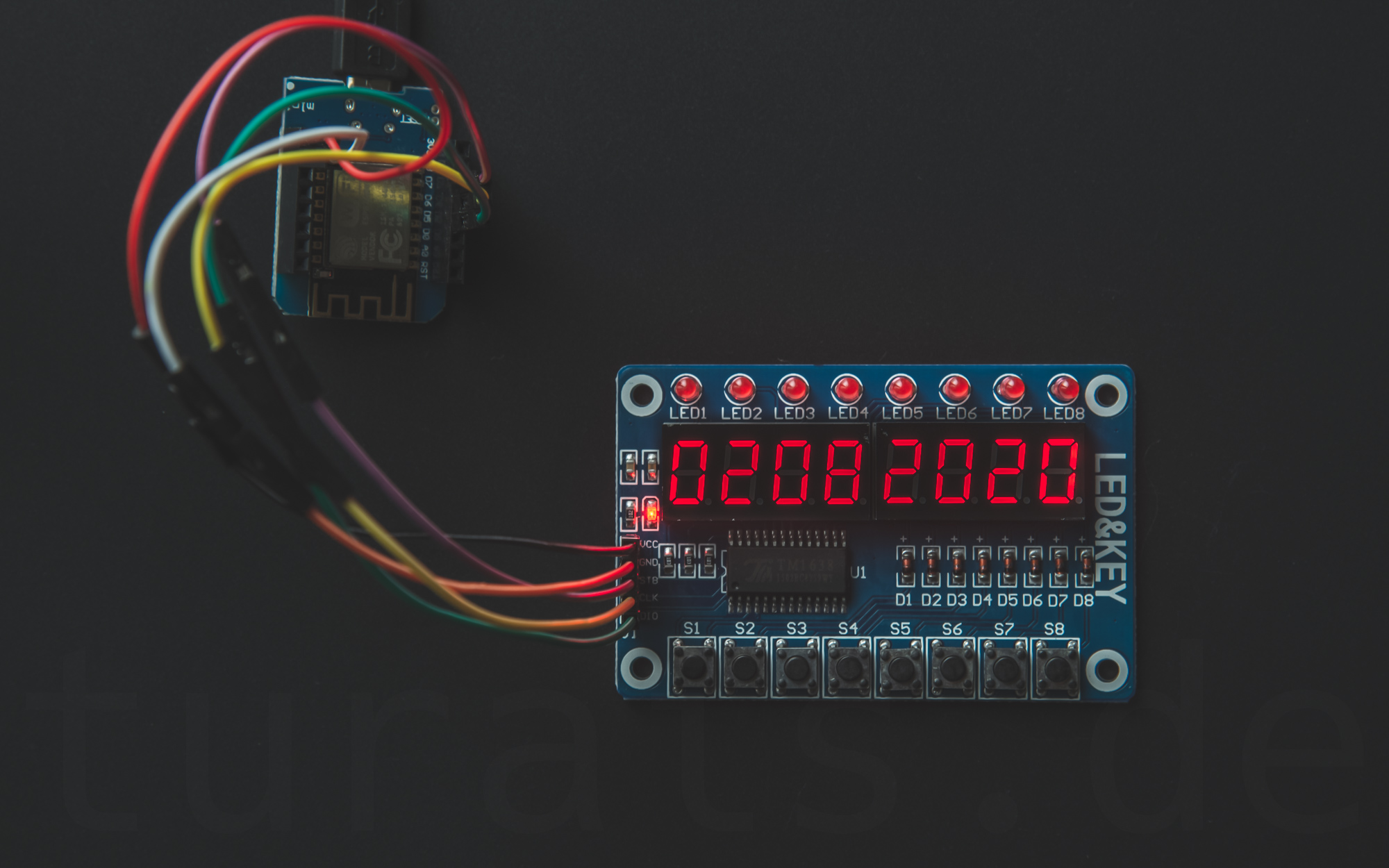# LED&KEY Module - TM1638

The LED&KEY Module you can find often on many sellers like Aliexpress or Ebay. The Module is based on the TM1638 Chip.

## OverviewLED&KEY

The LED&KEY Module is an ideal module if you want to test your code. Before testing it in a live environemnt, like when triggering valves, motors etc., you can easiliy test your code with this module. It has a user input with the 8 Buttons. Output can be shown with the 8 7-Segment displays. Also there are 8 LEDs available.

## ConnectionConnection of the WEMOS D1 Mini (ESP8266) with the LED&KEY Module

Wemos D1 Mini PIN LED&KEY Board PIN Remarks
3v3 VCC +3.3V
G GND Ground
D5 DIO Data I/O
D6 CLK Clock
D7 STB Standby

## Usage

The usage of the module is self-explanatory.

## Code

### Libraries

I forked the https://github.com/rjbatista/tm1638-library library because the original library would not work with `min`-functions.

The library works with the ESP8266.

Import the library:

``````#include <TM1638.h>
``````

Initalize the library with your pins.

``````///    ledkey_module(DIO, CLK, STB);
TM1638 ledkey_module(D5, D6, D7);
``````

### Examples

#### How do I power an LED?

With the tm1638-library from above you can do the following:

``````ledkey_module.setLEDs(led_state);
``````

where `led_state` is an byte representing the leds which are lighting up. For example:

Bit# 8 7 6 5 4 3 2 1
2^8 2^7 2^6 2^5 2^4 2^3 2^2 2^1
VAL: 0 0 0 0 0 1 1 0

This would be the value: `0x06` in hexadecimal. For convenience you can use an enumeration like so:

``````enum {
LED1 = 0u,
LED2 = 1u,
LED3 = 2u,
LED4 = 4u,
LED5 = 8u,
LED6 = 16u,
LED7 = 32u,
LED8 = 64u
};
``````

and then:

``````ledkey_module.setLEDs(LED1 | LED5 | LED7);
``````

If you want to set an LED without destroying the state before you have different options. Save the state in a global variable and then use an handy macro I wrote:

``````#define SET_BIT(A, i, val) \
do{ A &= ~(1u << i); A |= (val << i); } while(false)
``````

Using this macro is easy:

``````SET_BIT(led_state, number, val);
ledkey_module.setLEDs(led_state);
``````

where `number` is your led beginning with 0 and value either 0 or 1.

#### Writing something to the Display

To write a String to the 7-Segment is really easy. You can do it like so:

``````String str = "0123ABCD";
module.setDisplayToString(str);
``````To read the KeyPresses you have to constantly poll them an check the status. More on Polling here: Wikipedia: Polling

You can read the buttons with the library function: `getButtons()`. This call returns a byte in which every bit represents a button that is pressed. I recommend that you poll at least every 100ms. You can vary and experiment with this value a bit if you like. In your polling function it is recommended that you do this as little as possible.

I recommend something like this:

``````buttons = module.getButtons();
``````

This will return a `byte` representing the buttons pressed. For example if you press only button 1 this value would be `0x01`. If you want to check only one specific button press you can use the function with the enum below:

``````typedef enum {
BTN1 = 1,
BTN2 = 2u,
BTN3 = 4u,
BTN4 = 8u,
BTN5 = 16u,
BTN6 = 32u,
BTN7 = 64u,
BTN8 = 128u
} BTN;

bool get_pressed(const byte &state, const BTN check, ) {
byte btn_state = (state & check);
return btn_state / check;
}
``````

In your code you can now check for a specific button:

``````if(get_pressed(act_reading, BTN2)) {
//Button 2 was pressed
}
``````

For a more comprehensive example check out my GitHub example at: GitHub Example

#### Example on Github

You can find a working example on Github: turais

#### Blynk Example

https://github.com/turais/ledandkey_example